SQL Server排名函数和排名开窗函数

2018年11月16日 - MySQL

over(partition by colname)这样的分组排序。

Server中之row_number()、rank()、dense_rank()等排名函数，所有找到以下实现方式，在这简单记录转。

create table demo.Student (
ID int(11) NOT NULL AUTO_INCREMENT,
StuNo varchar(32) NOT NULL,
StuName varchar(10) NOT NULL,
StuAge int(11) DEFAULT NULL,
PRIMARY KEY (ID)
)
engine=innodb auto_increment=1 default charset=utf8 collate=utf8_general_ci;

insert into demo.Student(StuNo,StuName,StuAge) values('A001','小明',22);
insert into demo.Student(StuNo,StuName,StuAge) values('A005','小李',23);
insert into demo.Student(StuNo,StuName,StuAge) values('A007','小红',24);
insert into demo.Student(StuNo,StuName,StuAge) values('A003','小明',22);
insert into demo.Student(StuNo,StuName,StuAge) values('A002','小李',23);
insert into demo.Student(StuNo,StuName,StuAge) values('A004','小红',24);
insert into demo.Student(StuNo,StuName,StuAge) values('A006','小王',25);

select * from demo.Student;ROW_NUMBER

ROW_NUMBER函数是以上升进行直接排序，并且因为连续的逐条为各国一行数一个唯一的序号。（即行连）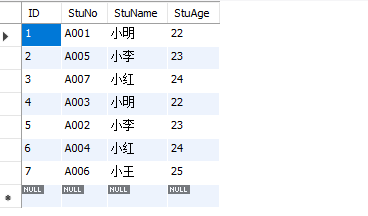1 -- 以下是根据 U_Pwd 这一列进行排名（升序）
2 select *,
3 '第'+convert(varchar,ROW_NUMBER() over(order by U_Pwd))+'名' RowNum
4 from UserInfoRANK 函数：并列排序，每当 order by

-- @row_number:=0，设置变量@row_number的初始值为0。
-- @row_number:=@row_number+1，累加@row_number的值。
select ID,StuNo,StuName,StuAge,@row_number:=@row_number+1 as row_number
from demo.Student a,
(
select @row_number:=0
) b
order by StuNo asc;

1 -- 以下是根据 U_Pwd 这一列进行排名（升序）
2 select *,
3 '第 '+convert(varchar,rank() over(order by U_Pwd))+' 名' RowNum
4 from UserInfo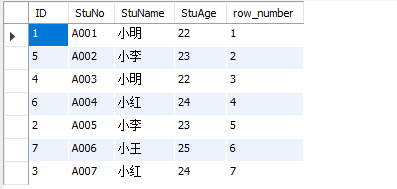DENSE_RANK

-- @StuAge:=null，设置变量@StuAge的初始值为null
-- @rank:=0，设置变量@rank的初始值为0
-- @inRank:=1，设置变量@inRank的初始值为1
-- if(@StuAge=StuAge,@rank,@rank:=@inRank)，指定排序列的值不变时，@rank的值不变；指定排序列的值变化时，@rank的值跳变为@inRank内部计数的值
-- @inRank:=@inRank+1，每一行自增1，用于实现内部计数
select t.ID,t.StuNo,t.StuName,t.StuAge,t.row_rank
from
(
select ID,StuNo,StuName,StuAge,if(@StuAge=StuAge,@rank,@rank:=@inRank) as row_rank,@inRank:=@inRank+1,@StuAge:=StuAge
from demo.Student a,
(
select @StuAge:=null,@rank:=0,@inRank:=1
) b
order by StuAge asc
) t;
1 -- 以下是根据 U_Pwd 这一列进行排名（升序）
2 select *,
3 '第 '+convert(varchar,DENSE_RANK() over(order by U_Pwd))+' 名' RowNum
4 from UserInfo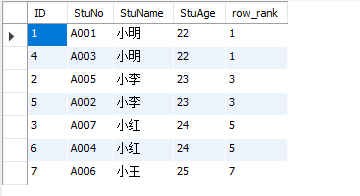NTILE

num_groups（指定的组数）。

-- @StuAge:=null，设置变量@StuAge的初始值为null
-- @rank:=0，设置变量@rank的初始值为0
-- if(@StuAge=StuAge,@rank,@rank:=@rank+1)，指定排序列的值不变时，@rank的值不变；指定排序列的值变化时，@rank的值自增1
select t.ID,t.StuNo,t.StuName,t.StuAge,t.row_rank
from
(
select ID,StuNo,StuName,StuAge,if(@StuAge=StuAge,@rank,@rank:=@rank+1) as row_rank,@StuAge:=StuAge
from demo.Student a,
(
select @StuAge:=null,@rank:=0
) b
order by StuAge asc
) t;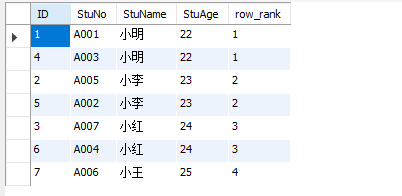colname)分组排名函数，按学生年龄(StuAge)分组排序。

1 -- 以下是根据 U_Pwd 这一列进行分组
2 select *,
3 '第 '+convert(varchar,NTILE(3) over(order by U_Pwd))+' 组' RowNum
4 from UserInfo
-- @StuAge:=null，设置变量@StuAge的初始值为null
-- @row_number:=0，设置变量@row_number的初始值为0
-- if(@StuAge=StuAge,@row_number:=@row_number+1,@row_number:=1)，指定排序列的值不变时，@row_number的值自增1；指定排序列的值变化时，@row_number的值等于1
select t.ID,t.StuNo,t.StuName,t.StuAge,t.row_number
from
(
select ID,StuNo,StuName,StuAge,if(@StuAge=StuAge,@row_number:=@row_number+1,@row_number:=1) as row_number,@StuAge:=StuAge
from demo.Student a,
(
select @StuAge:=null,@row_number:=0
) b
order by StuAge asc
) t;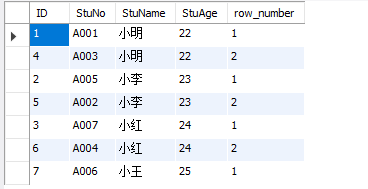PS：排名函数后面要产生 over() 子句。

MySQL提供了一个group_concat()函数，可以拿指定列的价拼成一个字符串，并可随指定排序方式并成字符，之间因此逗号隔开。如下示例：

select group_concat(StuNo order by StuNo asc) as column1,group_concat(StuNo order by ID asc) as column2
from demo.Student

ROW_NUMBER、DENSE_RANK、RANK、NTILE属于排名函数，OVER（）就是窗口函数。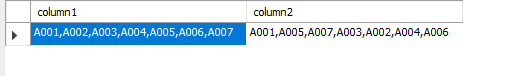BY就好本着数据开展分组，还可又返回基础实施的排列和聚合列。

select StuName,group_concat(StuNo order by StuNo asc) as column1,concat('"',group_concat(StuNo order by StuNo asc),'"') as column2
from demo.Student
group by StuName
order by StuAge

ODER BY 指定排名开窗函数的逐一。在排名开窗函数中务必利用ORDER BY语句。

PARTITION BY用于将结果集进行分组，开窗函数应用为各个一样组。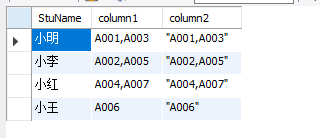1 -- 以下是先根据 U_Pwd 这一列进行分组，然后每一组再根据 U_Pwd 排序
2 select *,
3 '第'+convert(varchar,ROW_NUMBER() over(partition by U_Pwd order by U_Pwd))+'名' RowNum
4 from UserInfo1 -- 以下是先根据 U_Pwd 这一列进行分组，然后每一组再根据 U_Pwd 排序
2 select *,
3 '第'+convert(varchar,rank() over(partition by U_Pwd order by U_Pwd))+'名' RowNum
4 from UserInfo1 -- 以下是先根据 U_Pwd 这一列进行分组，然后每一组再根据 U_Name 排序
2 select *,
3 '第'+convert(varchar,rank() over(partition by U_Pwd order by U_Name))+'名' RowNum
4 from UserInfo1 -- 以下是先根据 U_Pwd 这一列进行分组，然后每一组再根据 U_Pwd 排序
2 select *,
3 '第'+convert(varchar,DENSE_RANK() over(partition by U_Pwd order by U_Pwd))+'名' RowNum
4 from UserInfoDENSE_RANK 再当各级一样组中展开排序，因为DENSE_RANK也是一视同仁排序，所以全部都是第一称。下面换个字段排序试试看。

1 -- 以下是先根据 U_Pwd 这一列进行分组，然后每一组再根据 U_Name 排序
2 select *,
3 '第'+convert(varchar,DENSE_RANK() over(partition by U_Pwd order by U_Name))+'名' RowNum
4 from UserInfo1 -- 以下是先根据 U_Pwd 这一列进行分组，然后每一组再根据 NTILE(3) 指定的组数分组，最后在根据 order by 子句指定的字段 U_Pwd 排序
2 select *,
3 '第'+convert(varchar,NTILE(3) over(partition by U_Pwd order by U_Pwd))+'名' RowNum
4 from UserInfoPS:在排序开窗函数中采用 PARTITION BY

http://www.cnblogs.com/jhxk/articles/2531595.html